Modulus

A numerical characteristic of various mathematical objects. Usually the value of a modulus is a non-negative real number, an element of, having certain characteristic properties, conditioned by properties of the setof objects under discussion. The notion of a modulus figures in various branches of mathematics, although sometimes under other names — absolute value; norm, etc. All of them, in essence, are generalizations of the idea of the absolute value of a real or complex number (but the term modulus usually means a generalization of special form). Here the function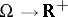turns out to be a morphism of some structure inonto one of the (algebraic) structures in, among which the most important ones are the order, the addition and the multiplication. In this connection the basic properties of the absolute value must be preserved (see below:)–)). In more abstract situations it is natural to use an ordered semi-ring instead of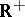(this conception of a modulus is satisfied by, for example, a measure, a capacity, a mass, etc.). Finally, the term modulus denotes numerical characteristics of other objects, such as, for example, the moduli of a plane domain, the modulus of an annulus, the moduli of a Riemann surface, and the modulus of continuity or smoothness (cf. Continuity, modulus of; Smoothness, modulus of) (and even moduli in the theory of elasticity (compression, shear)). However, in all these cases it is possible to introduce a value functionally depending on the modulus and more adequately reflecting the nature of the objects under discussion (for example, for a family of curves, the extremal length instead of the modulus).

Examples. 1) The modulus of an elementof a semi-ordered spaceis the number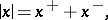where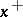() is the positive (negative) part of. Here, as for real numbers,)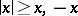;;)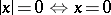(0 is the zero in).

2) The modulus of an elementof a separable pre-Hilbert space, in particular, a finite-dimensional vector space, is the number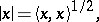whereis the inner product in. This is a norm inand thus)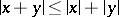;),a scalar.

3) The modulus of an elementof a locally compact skew-field is the number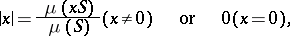whereis a Haar measure on the additive group ofandis a measurable subset. Here, as for numbers from,,,).

A generalization of this idea is the modulus of an automorphism.

4) The modulus of an endomorphismof a vector spaceover a field(a special case is the modulus of an automorphism) is the number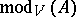, which turns out to be simply equal to, whereis the modulus of Example 3).